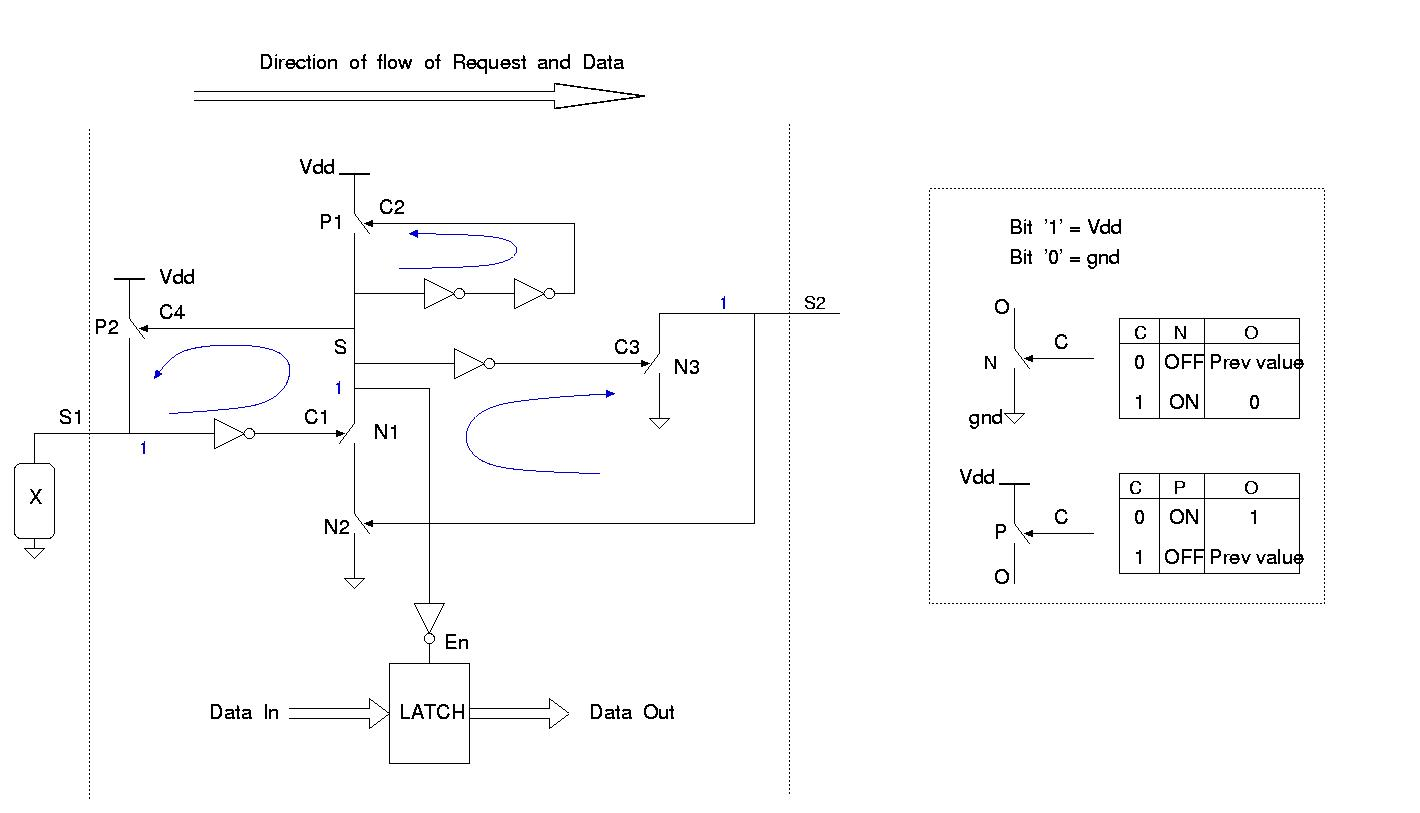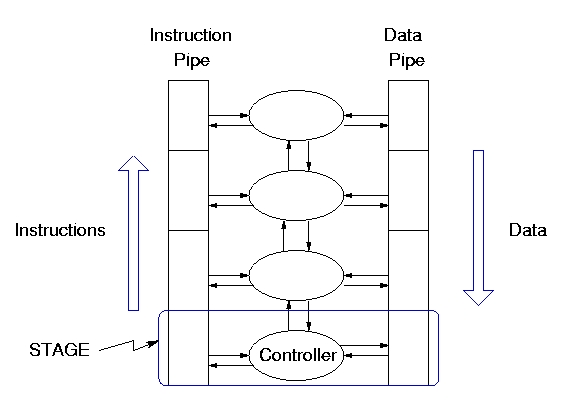# CS628 Projects

1. Given a process algebra description (text string) , the aim is to write a program that can decompose this process into elementary components like merge, arbiter, etc. Then, the original process and decomposed process should be checked for trace equivalence by using CWB.
2. Given a process algebra P, and a library L of process algebra, we intend to decompose the process P into the processes from the library L and elementary components like merge, arbiter, etc. Write a program to do this decomposition.
3. Given a proces algebra P and a set of production rules PRs, verify the correctness of PRs by feeding both PRs and the process algebra to CWB and check their trace equivalence. Represent the process P using PRs in verificationtool SPIN. Verify properties (which are evident from the process algebra) of the process using SPIN.
4. Given a proces algebra P and a set of production rules PRs, verify the correctness of PRs by feeding both PRs and the process algebra to CWB and check their trace equivalence. Represent the process P using PRs in verificationtool NuSMV. Verify properties (which are evident from the process algebra) of the process using NuSMV.
5. Given a process algebra, set of production rules PRs and some timing information about occurence of events in the process, obtain a simplified set ofproduction rules.

Example : Consider a process algebraic description P = (a? [] b? ) -> c? -> d! Suppose the timing information provided is a event b occurs between any two events on a. Then this information can be exploited to obtain a simpler set of production rules for process P.

6. Given an STG without choice (but with concurrency), the aim is to partition it structurally into approximately equal parts. You have to then verify their trace equivalence. Structural partitioning involves looking at the STG only (and not the corresponding STD) and break it up into smaller STGs. Thus, the input to your program will be an STG, and the output will be two or more STGs. Tool to be used in Petrify. Petrify allows you to represent STGs easily.
7. Given an STG without choice (but with concurrency), the aim is to partition it into two or more STGs based on the state-space traversal of the original STG. You have to then verify their trace equivalence. State-space based partitioning of an STG involves looking at the corresponding STD and then partitioning that STD into approximately equal smaller STDs. Thus, the input to your program will be an STG, and the output will be two or more STGs(not STDs). Tool to be used in Petrify.
8. Given an STG (with both choice and concurrency), you have to model it using the tool NuSMV. NuSMV is a Symbolic model checker. NuSMV allows to check finite state systems against specifications in the temporal logic CTL. You have to then verify interesting properties on the STG. Thus, the input to your program will be an STG, and output will be the modelling of that STG in the NuSMV input-language.
9. Given an STG, and some additional timing information, you have to simplify the given STG to another STG. The timing information will give the relative ordering between certain events. The simplification will be based on the state-space traversal of the original STG(i.e. based on the corresponding STD). You have to then use the tool CWB to verify whether all the traces of the simplified STG are contained in the set of traces of the original STG. Thus, the input to your program will be an STG, and the output will be a simplified STG.
10. Given an STG, and some additional timing information, you have to simplify the given STG to another STG. The timing information will give the relative ordering between certain events. The simplification will be based on the structure of the given STG (and not its state-space). You have to then use the tool Petrify to verify whether all the traces of the simplified STG are contained in the set of traces of the original STG. Thus, the input to your program will be an STG, and the output will be a simplified STG.11. GasP, shown in Fig. 1, is a fast asynchronous FIFO cell. Each cell is composed of the control circuit and data latching circuit. Several such cells can be cascaded to build linear and non-linear pipelines. If every switch (P/N) and the inverter shown in Fig. 1 has a time of operation as 1 time unit, then it takes 6 time units for transfering a request from S1 to S2, i.e. 6 gate delays for a GasP cell operation. The working of the GasP is as follows. Let, S1, S and S2 be referred to as conductors and C1, C2 and C3 the control signals for different switches. The operation of switch P and N is shown in the figure. When C is 1(0), N(P) is ON and O becomes 0(1). When C is 0(1), N(P) is OFF and O remains in its previous state. The initial values of the conductors S1, S and S2 are all 1 (shown in blue). Thus, P1, P2, N1 and N3 are OFF while N2 is ON. To begin data/request transfer, X(some circuit) pulls S1 low in the first stage of the FIFO. Following sequence of events happen after S1 is pulled low:

S1=0 -> N1 is turned ON -> N1 and N2 pull S low -> S=0.

After S becomes 0 following 4 things happen :

• S=0 -> C4=0 -> P2 is turned ON -> S1 is reset to 1. This turns off N1.
• S=0 -> C2=0 -> P1 is turned ON -> S is reset to 1.
• S=0 -> C3=1 -> N3 is turned ON -> is pulled to 0 which turns off N2.
• A pulse is generated on En due to 1 -> 0 -> 1 transition on S. This pulse enables a latch to latch the input data. The data is transferred from first stage to the next and the first stage is reset (as it becomes empty). It can now take on the next request. In a similar fashion request/data propogates through the entire pipeline when several GasP stages are connected.

Modeling GasP using NuSMV : Use NuSMV to model a GasP cell. The model should be such that it should allow you to build FIFOs by simply interconnecting several such cells. Finally, you should be able to verify certain interesting properties of GasP circuits in NuSMV. You may assume each gate delay to be of 1 time unit.

12. Modeling GasP using Kronos/Uppal : Use Kronos or Uppal to model a GasP cell. Kronos/Uppal allows you to verify real-time systems. Thus, your GasP cell model should take actual gate (switches and inverters) delays. The model should be such that it should allow you to build FIFOs by simply interconnecting several such cells. Finally, you should be able to verify interesting properties of GasP circuits.
13. Performance analysis of linear and non-linear GasP pipelines using NuSMV : Use NuSMV to model each GasP cell. You can model the GasP cell at a coarser level if required, i.e., you may not model each switch/inverter separately but can combine several gates/funtion as one element with appropriate delay. The GasP model should be such that it allows you to cascade several GasP cells to form different configurations such as simple linear pipelines, branch-and-join pipelines, circular (round robin) pipelines etc. For such configurations we would like to verify interesting properties for the pipelines. We would also like to do timing analysis of these configurations using NuSMV.
14. Timing analysis of XBM Machines :Write a program that takes as input XBM state transition diagram and generates as output, set of input vectors that can be used to perform timing analysis of the XBMs. A 0,1,X-simulator can be assumed to be available.
15. Automatic Decomposition of XBMs into communicating XBMs : Write a progam that takes as input XBM and decomposes it into two or more communicating XBMs.16. Modeling and Analysis of Counterflow pipeline processor (CFPP) architecture : Fig. 2 shows the basic CFPP architecture. Model one complete stage of CFPP using NuSMV or SPIN. The model should be such that it allows you to build a pipeline by interconnecting several such stages. You should be able to verify interesting properties of CFPP.
17. Optimization and Analysis of CFPP : There are conditions when CFPP can get blocked. We would like to alter the original CFPP and develop a new CFPP called as opti-CFPP which contains an extra data pipe(scratch pipe). The scratch pipe has a nice property that data is never stalled in this pipe and it continously keeps moving down. You need to develop the opti-CFPP architecture containing a scratch pipe and a new controller and argue about its performance versus performance of the original CFPP.
18. Modeling Timed XBMs : Use Kronos to model timed XBMs and verify interesting properties on it.
19. You have to implement the Grid communication protocol in a 3-D network using network sockets. You are well aware of this problem from your first homework, only that you have to extend it to 3-D and actually implement it using network sockets. You should then be able to actually do the timing analysis on the grid.